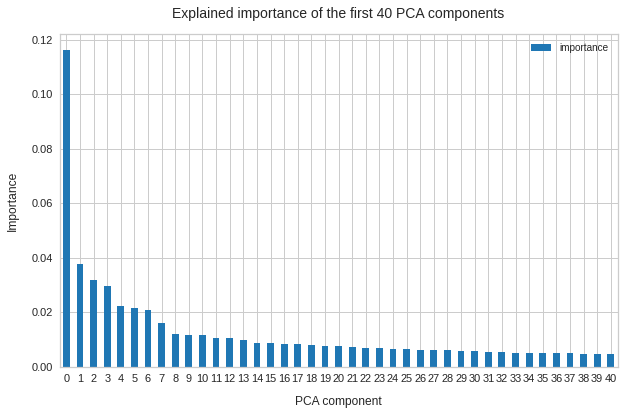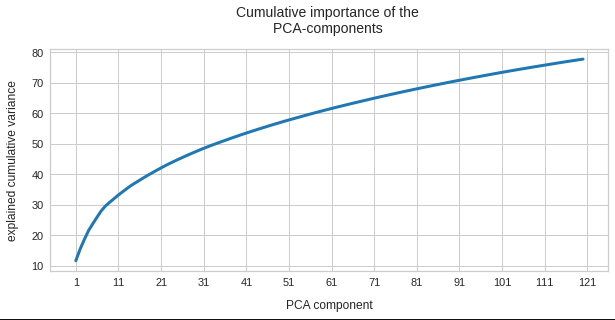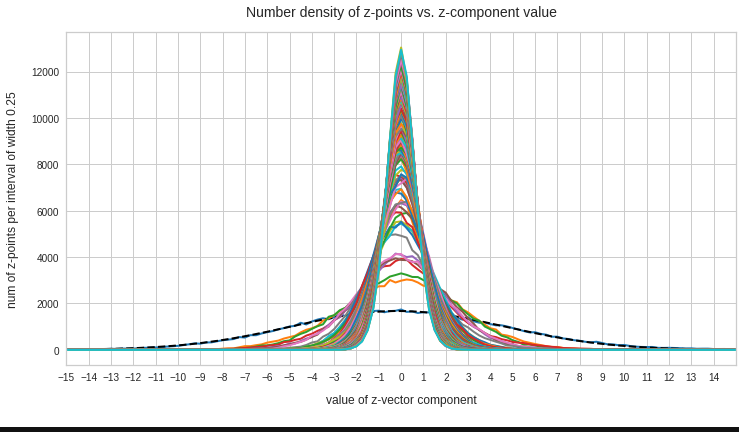# Autoencoders and latent space fragmentation – IX – PCA transformation of the z-point distribution for CelebA

This series of posts is about standard convolutional Autoencoders [AEs]. We wish to use the creative ability of an AE’s Decoder for the creation of human face images. We trained a relatively simple example AE on the CelebA data set. For the generation of new human face images we wanted to feed the Decoder with randomly created z-vectors in the AE’s latent space. As arbitrary latent vectors did not deliver reasonable images, we had a look at properties of the z-point distribution created in the latent space after training. And we found the z-vectors with end-points within this particular region indeed gave us some first reasonable images.

But during the first posts we also had to learn that it requires substantial effort to produce “randomly” created z-vectors which point to the coherent and confined latent space region populated by z-points for CelebA images. In addition we found that we obviously must fulfill complex correlation conditions between the latent vector components. In the last posts we have, therefore, investigated the structure of the latent space region populated for CelebA images a bit more closely. See:

Autoencoders and latent space fragmentation – VIII – approximation of the latent vector distribution by a multivariate normal distribution and ellipses

Autoencoders and latent space fragmentation – VII – face images from statistical z-points within the latent space region of CelebA

This gave us a somewhat surprising insight:

For our special case of human face images the density distribution of the z-points in the multidimensional latent space roughly resembled a multivariate normal distribution. The variables of this distribution were, of course, the components of the latent z-vectors (reaching from the origin of the latent space coordinate system to the z-points). The number density distributions for the values of the z-vector components could very well be approximated by Gaussian functions. In addition we found strong linear correlations between the z-vector components. This was completely consistent with diagonally oriented elliptic contour lines of the number density distribution for pairs of z-vector components in the respective coordinate planes. The angles between the ellipses’ axes and the axes of the (orthogonal) coordinate system varied.

In this post we test my thesis of a multivariate normal distribution in a different and more complicated way. If the number density distribution of the z-points for CelebA really is close to a multivariate normal distribution then we would expect that the contour lines of the 2-dimensional number density function for coordinate planes still are ellipses after a PCA transformation. Note that the coordinate planes then are planes of a new coordinate system (with orthogonal axes) moved and rotated with respect to the original coordinate system. We call the transformed coordinate system the “PCA coordinate system“. (It is clear the vector components must consistently be transformed with respect to the new coordinate axes.)

This test is much harder because asymmetries, imperfections and deviations from a normal distribution will become clearly visible. Nevertheless we shall see that the z-point distribution decomposes roughly in uncorrelated Gaussian number density (or probability) functions for the (transformed) components of the z-vectors – as expected.

Note that number density distributions can be interpreted as probability distributions after a proper normalization. To understand the results and implications of this post some basic knowledge about multivariate normal probability density distributions in multidimensional spaces is required. Transformation properties of such distributions are important. In particular the effect of a PCA or SVD transformation, which diagonalizes the Pearson correlation matrix, on a multivariate normal distribution, should be familiar: In the moved and rotated coordinate system original linear correlations between vector components disappear. The coordinate axes of the transformed coordinate system get aligned to the eigenvectors (and main axes) of the probability density distribution. In addition elliptic contour lines prevail and the main axes of the ellipses get aligned with the axes of the PCA coordinate system.

# Imperfections in the multivariate normal distribution and their consequences for PCA

In our case the latent space had 256 dimensions. The real number density functions for the values of the (256) latent vector components did not always show the full symmetry of their Gaussian fits. Deviations appeared near the center and at the flanks of the individual distributions. Still we got convincing overall ellipses in contour plots of the probability density for selected pairs of components. But there were also relatively many points which lay in regions outside the 2 to 3 sigma confidence ellipses for these 2-dimensional distributions – and the distribution of the points there seemed to violate the elliptic symmetry.

A perfect multivariate normal distribution (with only linear correlations between its variables) decomposes into (seemingly) uncorrelated normal distributions for the new variables, namely the latent vector components in the transformed coordinate system of the latent space. The coordinate transformation corresponds to a diagonalization of the Pearson correlation matrix via finding orthogonal eigenvectors. So we should not only get ellipses after the PCA transformation. The main axes of the resulting ellipses should also be aligned with the coordinate axes of the new translated and rotated PCA coordinate system. (This is a standard feature of “uncorrelated” Gaussians).

Note that the de-correlation is a pure coordinate effect which does not eliminate the dependencies of the original variables. The new coordinates and the respective variables do not have the same meaning as the old ones. We “only” change perspectives such that the mathematical description of the multivariate distribution gets simpler. However, the ratios of the axes of the new ellipses depend on the axis-ratios of the old ellipses in the original standard coordinate system of the latent space.

When we translate and rotate a coordinate system to diagonalize the Pearson matrix of an imperfect multivariate normal distribution the coordinate axes may afterward not fully align with the main axes of the distribution’s inner core – and then we might get clearly visible asymmetries in z-point projections to coordinate planes. Note that points outside the inner core have a relatively large impact on the PCA transformation and especially on the rotation of the new axes with respect to the old ones.

# Reduction of outer z-point contributions

Outer z-points often correspond to CelebA images with strong variations in the background. As these z-points have a large distance from the center of the distribution they have a heavy impact on a PCA transformation to eigenvectors of the Pearson matrix. Therefore, I eliminated some outer points from the distribution. I did this by deleting points with coordinate values smaller or bigger than a symmetric threshold in the outer flanks of the 256 Gaussian distributions – e.g.:

z_j < mu_j – fact * FHWM and z_j > mu_j + fact * FHWM.

With FHWM meaning the full width at the half maximum and mu_j being the center of the approximate Gaussian for the j-th vector component of the z-vectors. I tried multiple values of fact between 1.7 and 1.25.

One has to be careful with such an elimination process. Even though we set our limits beyond the 2 σ-level of the individual Gaussians ahead of the transformation, a step-wise consecutive elimination for all components reduces the number of surviving vectors significantly for small values of fact. The plots below correspond to a value of fact = 1.7 (with one discussed exception). This reduced the number of available vectors from originally 170.000 used during the AE’s training to 160.000. From this distribution a random sub-selection of 80,000 z-points was taken to perform the PCA transformation. I call the resulting sample of z-points the “reduced distribution” below. The results did not change much for a sub-selection of more points. The second reduction saved me some CPU-time, however.

# PCA transformation of the z-point distribution – explained variance and cumulative importance

Below you see the explained variance ratio of the first 40 and the cumulative importance of the first 120 main components after a PCA transformation of the reduced z-point distribution. (The PCA-algorithm was based on the SVD-algorithm, as provided by the sklearn-package.)We see that we need around 120 PCA components to explain 80% of the variance of the original z-point data. The contribution of the first 15 components is significant, but it is NOT really dominant. Interesting is also the step-wise decline of the first 10 components in a not so smooth curve.

I have in addition checked that the normalized Pearson correlation coefficient matrix was perfectly diagonalized. All off-diagonal values were smaller than 2.e-7 (which is consistent with error propagation) and the diagonal elements were 1.0 with an accuracy better than 8 eight digits after the decimal point. As it should be.

# Gaussians per PCA-vector component?

We expect Gaussian number density functions for each of the z-vector components after the PCA coordinate transformation. The following line plots show the number density distributions (colored line) and a related Gaussian fit (dashed lines) per named vector-components after PCA-transformation. The first plot gives an overview over 120 PCA components:We see that all distributions look similar to Gaussians. In addition the center of the distributions for all components are very close to or coincide with the origin of the PCA coordinate system. This is something we would expect for an original multivariate normal distribution before the PCA transformation to a new moved and rotated coordinate system: An affine transformation between coordinate systems with orthogonal axes maps Gaussians to Gaussians.

We see from these plots that component 3 shows values at the distributions’s flanks well above the values of its Gaussian fit. This may lead to deformed ellipses. In addition component 7 shows a slight asymmetry – probably triggering deviations both at the center and outer regions. Similar effects can be seen for other higher components – but not so pronounced. The chosen sampling interval (0.5) in the new coordinates did smear out small asymmetric wiggles in all distributions.

# Ellipses?

Calculating approximate contour lines is CPU intensive. On my presently available laptop I had to focus on some selected examples for pairs of components of z-vectors in the transformed coordinate system. Experiments showed that the most critical components with respect to asymmetries were 2, 3, 7, 8.

Let us first look at combinations of the first 9 PCA components (0) with other components in the range between the 10th and 120th PCA component. Just scroll down to see more images:

The fatter lines represent confidence ellipses derived both from correlation data, i.e. elements of the normalized correlation coefficient matrix, and properties of the Gaussian distributions. See the last blog for details on the method of getting confidence ellipses from data of a probability distribution.

# Ellipses for density distributions in coordinate planes for pars of the most important PCA components

Before you think that everything is just perfect we should focus on the most important 10 PCA components and their mutual pair-wise density distributions. We expect trouble especially for combinations with the components 2, 3 and 7.

Component 0 vs other components < 10

We see already here that asymmetries in the density distributions which appear relatively small in the gaussian-like curves per component leave their imprint on the elliptic curves. The contour lines are not always centered with respect to the overall confidence ellipses.

Component 1 vs other components < 10

Component 2 vs other components < 10

The plot for components 2 and 3 was based on fact = 1.35 instead of fact = 1.7. See a section below for more information.

Component 3 vs other components < 10

Component 4 vs other components < 10

Component 5 vs other components < 10

Component 6 vs other components < 10

Component 7 vs other components < 10

Component 8 vs other components < 10

Component 9 vs other components < 10

# Ellipses for other seected component pairs

The z-point distribution is rather close to a multivariate normal distribution, but it is not perfect. The PCA transformation revealed clearly that the center of the overall z-point distribution does not completely coincide with the centers of the individual density distributions for the z-vector components. And not all individual curves are fully symmetrical. Some plots show the expected ellipses, but not fully centered. We also see signs of non-linear correlations as not all ellipses show a complete alignment with the axes of the PCA coordinate system.

The impact of outer z-points becomes obvious when we compare plots for the component pair (2, 3) for different values of fact. The plots are for fact = 1.35, 1.45, 1.55, 1.7 and fact = 1.8 (in this order from left to right and downward). (Regarding z-point elimination the fact-values correspond to 122000, 138000, 149000, 159000 and 164000 remaining z-points.)

The flips in the left-right orientation between some of the plots can be ignored. They depend on random aspects of the transformations and plotting routines used.

We see that z-points (for some some strange images) which have a large distance from the center of the distribution have a major impact on the central density distribution. 10% of the outer points influence the orientation of the central ellipses by their coordinates – although these outer points are not part of the inner core of the distribution.

BUT: Overall we find the effect we wanted to see. The elliptic contours are clearly visible and most of them are aligned with the axes of the PCA coordinate system and the main axes of the confidence ellipses for the z-point distribution in the PCA coordinate system. The inner core is aligned with the axes of the coordinate system even for the critical PCA components 2 and 3, when we omit between 12% and 25% of the points (outside a 3 σ-level).

This means: The PCA transformation confirms that an inner core of the z-point distribution is well described by a multivariate normal distribution – with linear correlations between the number density distributions for various components.

This is an interesting finding by itself.

# Conclusion

The last and the present post of this series have shown that a convolutional AE maps human face images of the CelebA data set to a multivariate normal distribution in its multidimensional latent space. Although this is interesting by itself we must not forget our ultimate goal – namely to generate random z-vectors which should deliver us reasonably human face images by the AE’s Decoder. But the results of our analysis provide solid criteria to generate such vectors fulfilling complex correlation conditions.

We can now define statistical generation methods which restrict the components of our aspired random z-vectors such that the resulting z-points lie within regions surrounded by confidence ellipses of the multivariate normal distribution. One such method is the topic of the next post.

# Links to other posts of this series

Autoencoders and latent space fragmentation – VIII – approximation of the latent vector distribution by a multivariate normal distribution and ellipses

Autoencoders and latent space fragmentation – VII – face images from statistical z-points within the latent space region of CelebA

Autoencoders and latent space fragmentation – VI – image creation from z-points along paths in selected coordinate planes of the latent space

Autoencoders and latent space fragmentation – V – reconstruction of human face images from simple statistical z-point-distributions?

Autoencoders and latent space fragmentation – IV – CelebA and statistical vector distributions in the surroundings of the latent space origin

Autoencoders and latent space fragmentation – III – correlations of latent vector components

Autoencoders and latent space fragmentation – II – number distributions of latent vector components

Autoencoders and latent space fragmentation – I – Encoder, Decoder, latent space